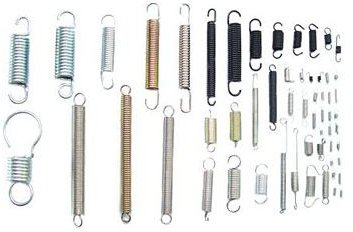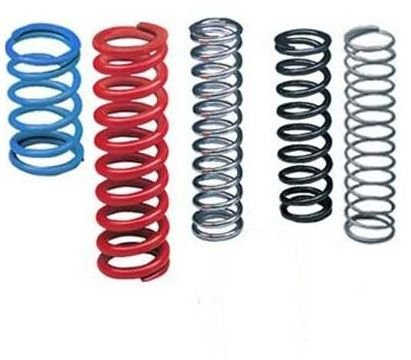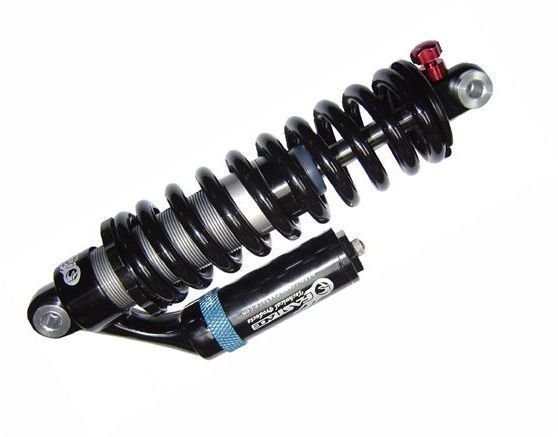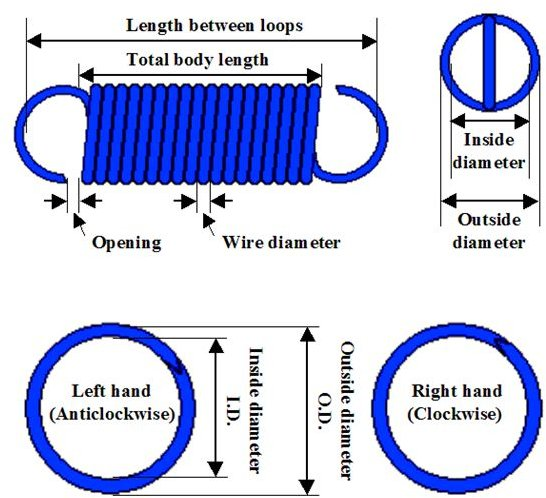# All Machine Parts – Compression and Tension Coil Springs Overview

## What is a Spring

A spring is a mechanical device that is used to store energy in it (as potential energy) and release it up at a later stage as kinetic energy. Elasticity is one phenomenon that can be related to the working of springs. According to this property of materials, a force (to a certain level) when applied to it tries to deform the same which vanishes and the material regains its original position once the force is removed. This property of materials suggested that the springs that are made of elastic materials would be more efficient and last longer.

## Most Popular Springs

Though there are various designs of the springs, the most popular and the common ones that have found their way into our daily activities are the coil springs. The elastic material is made into coils of predetermined number and length. Coil springs are of two types categorized according to their functionality viz. ‘Tension’ springs and ‘Compression’ springs.

Compression springs decrease in length when a force is applied and they function under axial loads, while the tension springs increase in length when an axial force is applied. Compression springs are found, for example, in shock absorbers of automobiles and tension spring can be found in a spring balance.

Springs of both these types are found in many places where a high load is involved. Primarily used to cushion the impact of the load, these springs can also be used to multiply the force applied like the case of an air gun. One major disadvantage of the compression springs is the prolonged oscillations when a load is applied. So separate members like dampers need to be provided to curb this oscillation which has a negative impact on the functioning of the spring.

## Tension and Compression Springs## Hook’s Law

Robert Hooke in the 17th century formulated a universal law related to the springs which is still in use in classical physics. According to Hooke, the force exerted by a spring (tensile or compression) when loaded is directly proportional to the distance moved from its equilibrium length. This formulated ways to calculate the ‘spring constant’ which was a constant value for a spring and the magnitude was evaluated by dividing the force by the displacement.

## Spring Rate (Spring Consant)

The characteristic of the spring depends on the material from which it is made. They are generally made up of various materials like High carbon wire, steel alloys, stainless steel and non ferrous metals. Spring materials should generally have high tensile strength if the application involves fluctuations in the load. Other factors that influence coil spring constant (or coil spring rate) are the number of turns, length of spring, and, most important wire diameter, which is connected to the spring rate with a factor of 4. This means that if throughout your coil spring rate calculation you multiply the wire diameter by two the spring becomes 16 times stronger!

## Unlimited Life?

Though ideally infinite, actual life of a spring depends on number of factors like material, number of cycles, temperature range of operation, etc. When properly chosen and designed it can continuously undergo infinite cycles. One such example is that of the valve springs in the engine which undergo more than a million cycles daily. These types of springs do not fail until exposed to a very high temperature altering its tensile strength, or a high load creating a stress above the elastic region where the material and the spring begins to lose its elasticity to enter the plastic region.